# Medicine

We test medicine on 6 patients. For all drug doesn't work. If the drug success rate of 20%, what is the probability that medicine does not work?

Correct result:

p =  26.21 %

#### Solution: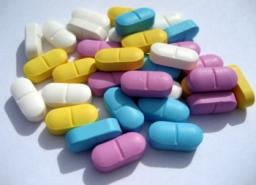We would be pleased if you find an error in the word problem, spelling mistakes, or inaccuracies and send it to us. Thank you!Tips to related online calculators
Would you like to compute count of combinations?

## Next similar math problems:

• Double probabilityThe probability of success of the planned action is 60%. What is the probability that success will be achieved at least once if this action is repeated twice?
• ShootersIn army regiment are six shooters. The first shooter target hit with a probability of 49%, next with 75%, 41%, 20%, 34%, 63%. Calculate the probability of target hit when shooting all at once.
• Utopia IslandA probability of disease A on the island of Utopia is 40%. A probability of occurrence among the men of this island, which make up 60% of all the population (the rest are women), is 50%. What is the probability of occurrence of A disease among women on Ut
• TestThe teacher prepared a test with ten questions. The student has the option to choose one correct answer from the four (A, B, C, D). The student did not get a written exam at all. What is the probability that: a) He answers half correctly. b) He answers al
• FamilyWhat is the probability that a family with 7 childrens have: exactly 5 girls? 7 girls and 0 boys? Consider the birth probability of a girl is 48.69% and boy 51.31%.
• All use computerIt is reported that 72% of working women use computers at work. Choose 3 women at random, find the probability that all 3 women use a computer in their jobs.
• The testThe test contains four questions, and there are five different answers to each of them, of which only one is correct, the others are incorrect. What is the probability that a student who does not know the answer to any question will guess the right answer
• Probability of malaria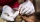A survey carried out at a certain hospital indicates that the probability that a patient tested positive for malaria is 0.6. What is the probability that two patients selected at random (i) one is negative while the other tested positive? (i) both patient
• The manufacturer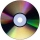The manufacturer found that 3% of the plates produced had a malfunction. Of the compliant, 75% are first and 25% second. What is the probability of producing first and second class plates?
• Six questions testThere are six questions in the test. There are 3 answers to each - only one is correct. In order for a student to take the exam, at least four questions must be answered correctly. Alan didn't learn at all, so he circled the answers only by guessing. What
• The universityAt a certain university, 25% of students are in the business faculty. Of the students in the business faculty, 66% are males. However, only 52% of all students at the university are male. a. What is the probability that a student selected at random in the
• Left handedIt is known that 25% of the population is left-handed. What is the probability that there is a maximum of three left-handers at a seminar where there are 30 participants?
• Math testObelix filled a mathematical test in which he answered 25 questions. For every correct answer, he received 5 points, for each bad answer he had 3 points deducted. Obelix gained 36% of all points in the test. How many questions did he solve correctly?
• Bureau of Labor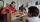Bureau of Labor is a state institution that provides mike and the rest for their so-called clients. The mission of the Bureau of Labor is spend taxpayer money to provide relaxation and benefits to those who do not want to work. Popularly speaking sense th
• Alarm systems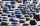What is the probability that at least one alarm system will signal the theft of a motor vehicle when the efficiency of the first system is 90% and of the independent second system 80%?
• VAT lottery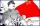Father earns 1088 euro a month, mother 925 euro per month. Calculate what amount they paid as value added tax (VAT) to the government if the VAT rate is 20%. Assume that the family will spend their entire monthly income.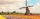How large must the group of people be so that the probability that two people have a birthday on the same day of the year is greater than 90%?